Ex 4.5

Chapter 4 Class 12 Determinants
Serial order wise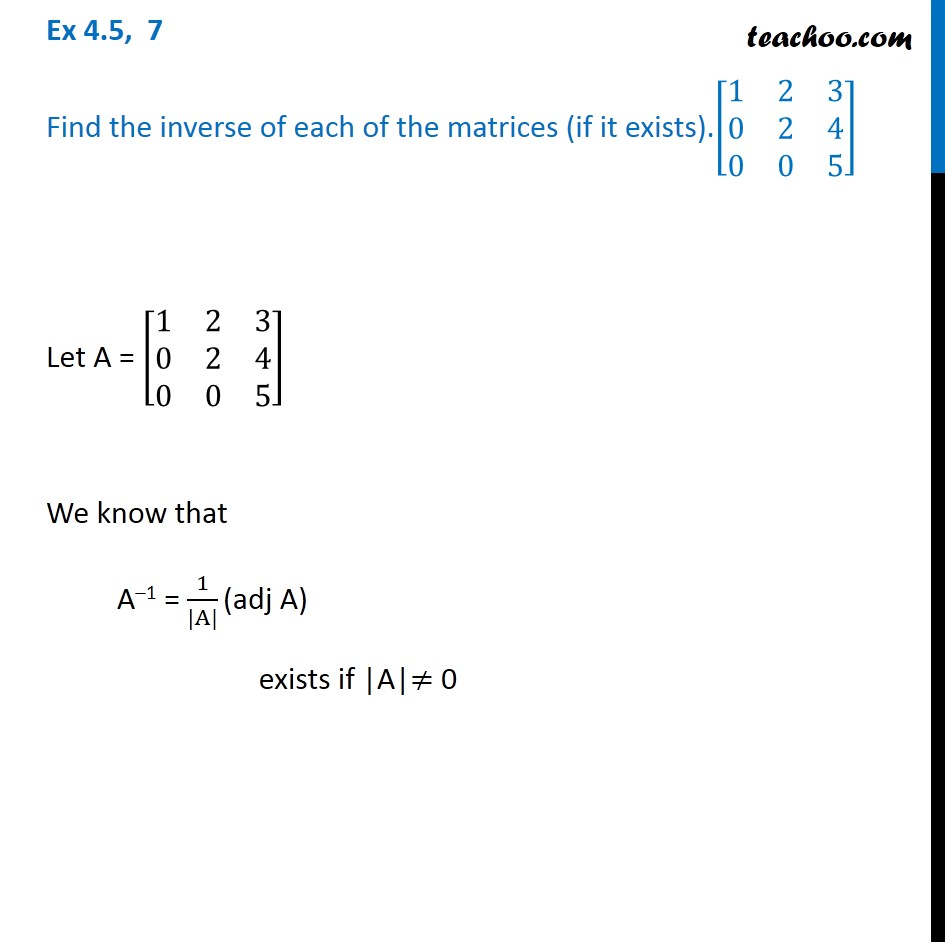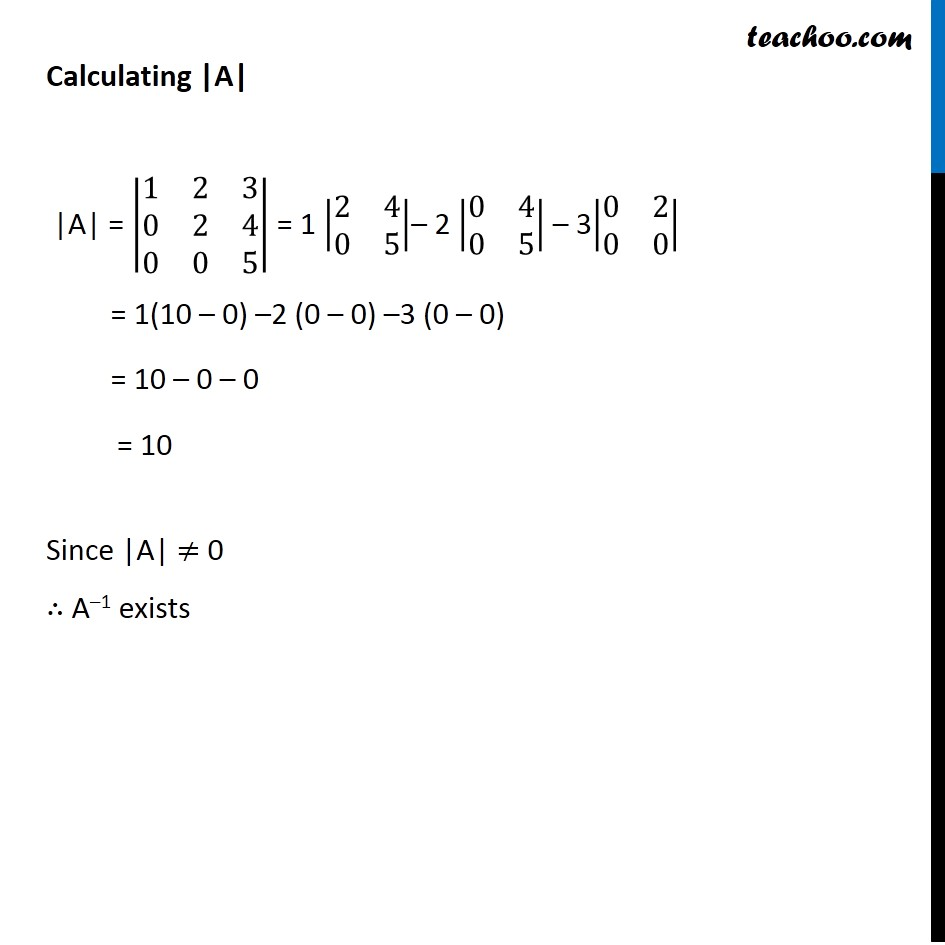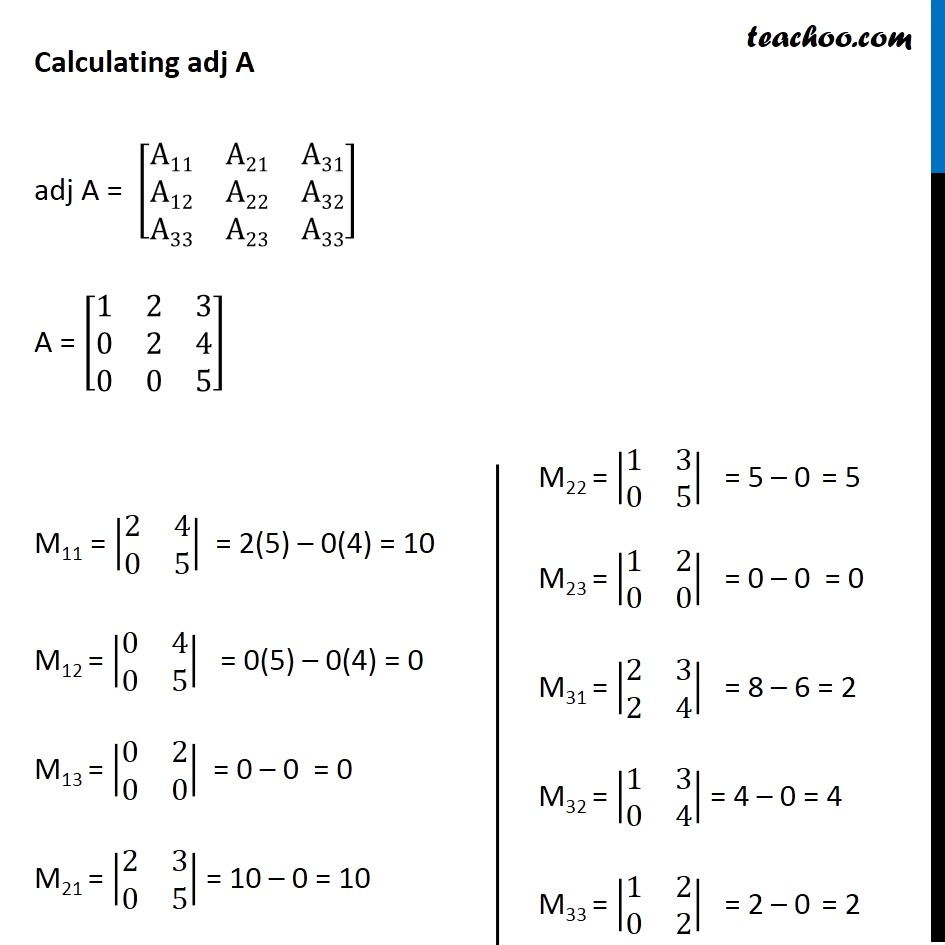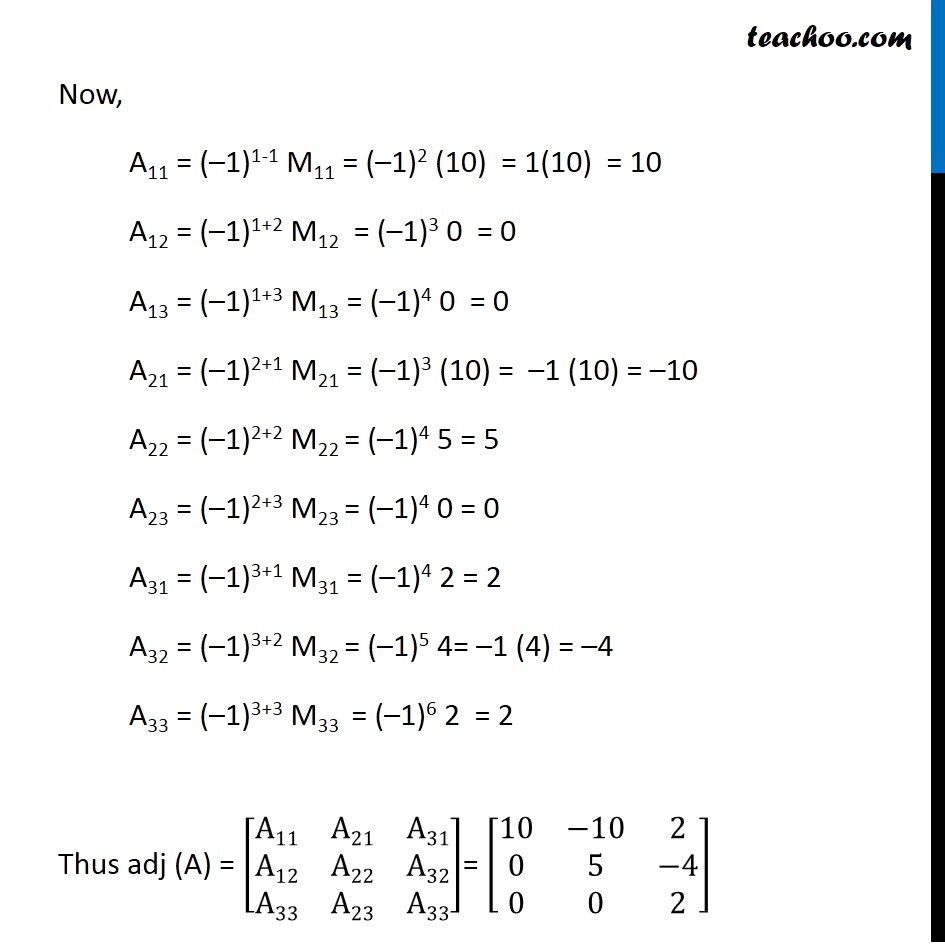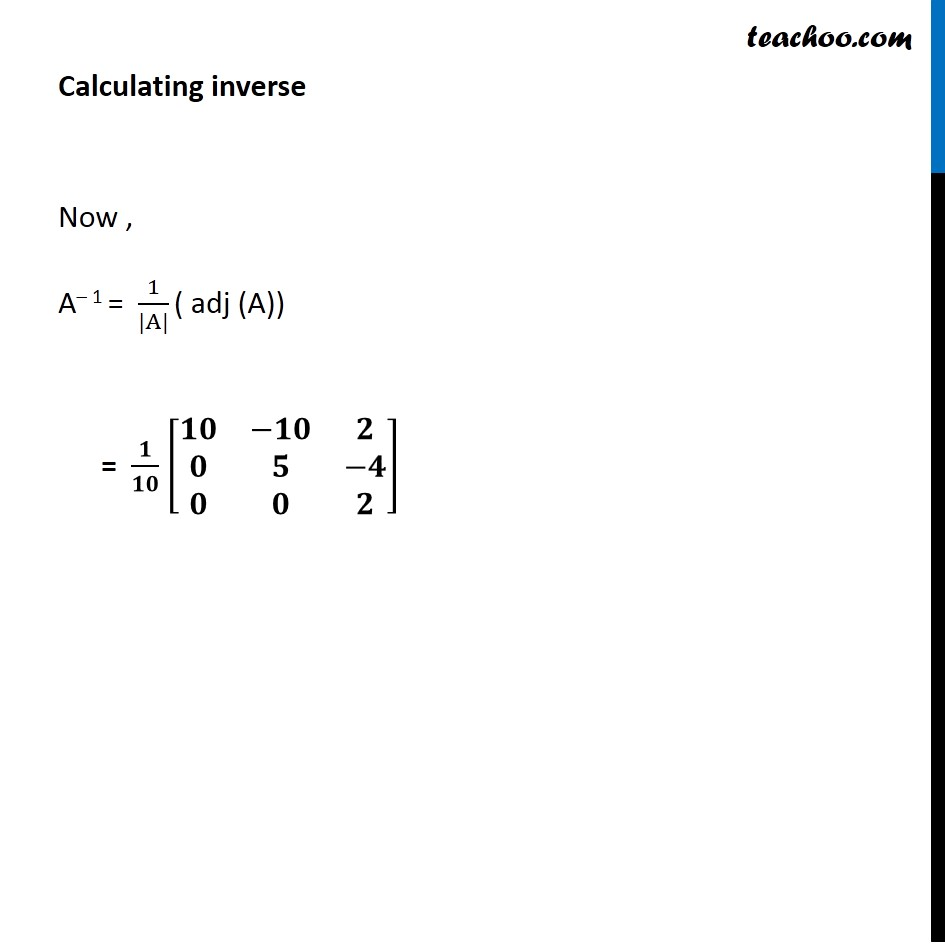Solve all your doubts with Teachoo Black (new monthly pack available now!)

### Transcript

Ex 4.5, 7 Find the inverse of each of the matrices (if it exists).[■8(1&2&3@0&2&4@0&0&5)] Let A = [■8(1&2&3@0&2&4@0&0&5)] We know that A–1 = 1/(|A|) (adj A) exists if |A|≠ 0 Calculating |A| |A| = |■8(1&2&3@0&2&4@0&0&5)| = 1 |■8(2&4@0&5)|– 2 |■8(0&4@0&5)| – 3|■8(0&2@0&0)| = 1(10 – 0) –2 (0 – 0) –3 (0 – 0) = 10 – 0 – 0 = 10 Since |A| ≠ 0 ∴ A–1 exists Calculating adj A adj A = [■8(A11&A21&A31@A12&A22&A32@A33&A23&A33)] A = [■8(1&2&3@0&2&4@0&0&5)] M11 = |■8(2&4@0&5)| = 2(5) – 0(4) = 10 M12 = |■8(0&4@0&5)| = 0(5) – 0(4) = 0 M13 = |■8(0&2@0&0)| = 0 – 0 = 0 M21 = |■8(2&3@0&5)| = 10 – 0 = 10 M22 = |■8(1&3@0&5)| = 5 – 0 = 5 M23 = |■8(1&2@0&0)| = 0 – 0 = 0 M31 = |■8(2&3@2&4)| = 8 – 6 = 2 M32 = |■8(1&3@0&4)| = 4 – 0 = 4 M33 = |■8(1&2@0&2)| = 2 – 0 = 2 Now, A11 = (–1)1-1 M11 = (–1)2 (10) = 1(10) = 10 A12 = (–1)1+2 M12 = (–1)3 0 = 0 A13 = (–1)1+3 M13 = (–1)4 0 = 0 A21 = (–1)2+1 M21 = (–1)3 (10) = –1 (10) = –10 A22 = (–1)2+2 M22 = (–1)4 5 = 5 A23 = (–1)2+3 M23 = (–1)4 0 = 0 A31 = (–1)3+1 M31 = (–1)4 2 = 2 A32 = (–1)3+2 M32 = (–1)5 4= –1 (4) = –4 A33 = (–1)3+3 M33 = (–1)6 2 = 2 Thus adj (A) = [■8(A11&A21&A31@A12&A22&A32@A33&A23&A33)]= [■8(10&−10&2@0&5&−4@0&0&2)] Calculating inverse Now , A– 1 = 1/(|A|) ( adj (A)) = 𝟏/𝟏𝟎 [■8(𝟏𝟎&−𝟏𝟎&𝟐@𝟎&𝟓&−𝟒@𝟎&𝟎&𝟐)]